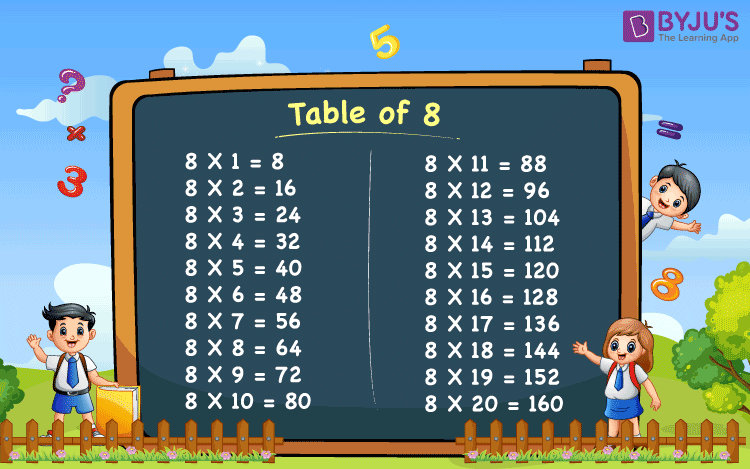# Table of 8

Table of 8 is one of the most crucial maths tables as most of the questions in primary classes are based on it. The 8 times table can be a bit troublesome for some students as the values are a little difficult to remember and are hard to calculate for the school students.

## Table of 8 ChartTable of 8 is given here in both tabular and image format. The 8 multiplication table given here can help the students to check the values quickly and memorise them better. Students can also download 8 times table image and keep revising the values periodically.

## What is 8 times table?

We can write the 8 table with the help of the repeated addition of 8. So, the multiplication table of 8 can be represented as given below:

8 × 1 = 8 = 8

8 × 2 = 8 + 8 = 16

8 × 3 = 8 + 8 + 8 = 24

8 × 4 = 8 + 8 + 8 + 8 = 32

8 × 5 = 8 + 8 + 8 + 8 + 8 = 40

8 × 6 = 8 + 8 + 8 + 8 + 8 + 8 = 48

8 × 7 = 8 + 8 + 8 + 8 + 8 + 8 + 8 = 56

8 × 8 = 8 + 8 + 8 + 8 + 8 + 8 + 8 + 8 = 64

8 × 9 = 8 + 8 + 8 + 8 + 8 + 8 + 8 + 8 + 8 = 72

8 × 10 = 8 + 8 + 8 + 8 + 8 + 8 + 8 + 8 + 8 + 8 = 80

As this lead to multiple additions, we generally use multiplication to write the tables in maths.

## Multiplication Table of 8

The below table shows the 8 times table from 1 to 20. These are also called the first 20 multiples of 8.

 8 × 1 = 8 8 × 2 = 16 8 × 3 = 24 8 × 4 = 32 8 × 5 = 40 8 × 6 = 48 8 × 7 = 56 8 × 8 = 64 8 × 9 = 72 8 × 10 = 80 8 × 11 = 88 8 × 12 = 96 8 × 13 = 104 8 × 14 = 112 8 × 15 = 120 8 × 16 = 128 8 × 17 = 136 8 × 18 = 144 8 × 19 = 152 8 × 20 = 160

### How to Read Table of 8?

Read the eight times table from 1 to 10 as provided below.

• One time eight is eight
• Two times eight is sixteen
• Three times eight is twenty-four
• Four times eight is thirty-two
• Five times eight is forty
• Six times eight is forty-eight
• Seven times eight is fifty-six
• Eight times eight is sixty-four
• Nine times eight is seventy-two
• Ten times eight is eighty

For check more maths table and learn different maths concepts easily, stay tuned with BYJU’S.

Get More Maths Tables:-

Test your Knowledge on Table of 8